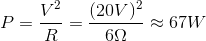# AP Physics C: Mechanics : Calculating Circuit Power

## Example Questions

### Example Question #20 : Electric Circuits

A simple circuit contains two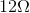resistors in parallel, connected to a 20V source. What power is being provided by the source to the circuit?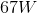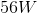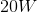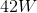Explanation:

The power supplied to the circuit can be calculated using the equation: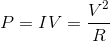To use this equation, we need to find the equivalent resistance of the circuit. Use the equation for equivalent resistance in parallel: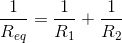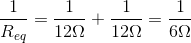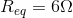Now that we have the resistance and the voltage, we can solve for the power.